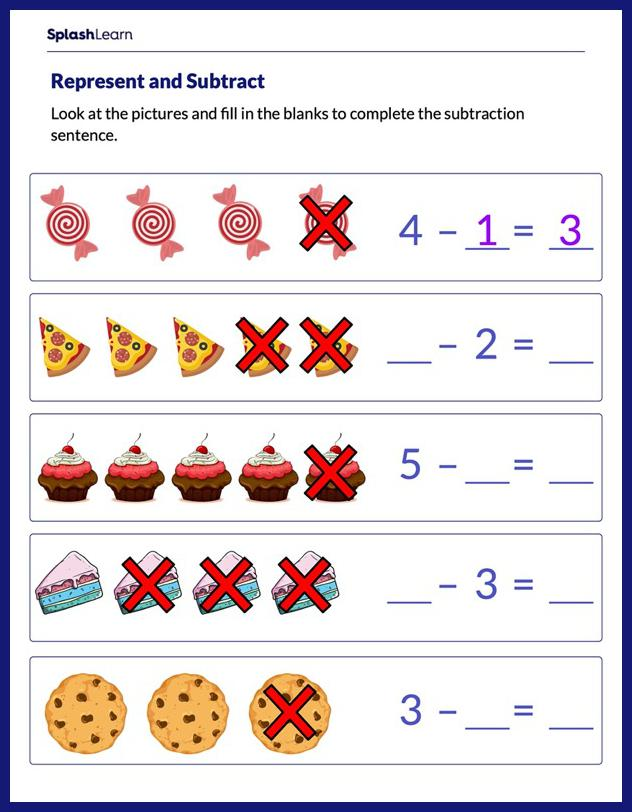# Represent and Solve Subtraction Equations Worksheet

Home > Represent and Solve Subtraction EquationsKids often develop misconceptions about concepts in mathematics, including subtraction. It is important to help them get over these misconceptions. The worksheet requires students to work with a set of problems on subtraction and use their conceptual understanding to find the missing numbers in math sentences. Students will work with numbers within 10.